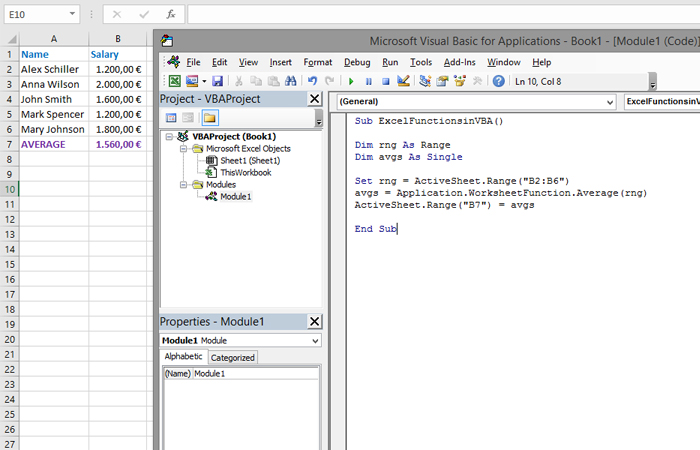# Excel functions in VBA

The VBA language can use two types of functions: those inherent to it and functions that already exist in Excel. Although VBA has a large number of functions in Excel there are even more of them. And since we don’t want,  as the saying goes, to „invent the hot water“ it’s often better to use one of the existing functions to finish the job. This is accomplished using the Application.WorksheetFunction method that allows us to call any available Excel function.

How to perform this I will show in the example where we should calculate the average salary for a group of workers. Before starting the executable part of the program, we first need to declare two variables. The first represents the range, and the second should contain the result of the function. Then, the object representing the range is assigned the range whose average we calculate (B2:B6), further we invoke the AVERAGE function using the WorksheetFunction method. Finally, we enter the result into the corresponding cell (B7). When completed, a program should look like this:

Sub ExcelFunctionsinVBA()

Dim rng As Range
Dim avgs As Single
Set rng = ActiveSheet.Range(“B2:B6”)
avgs = Application.WorksheetFunction.Average(rng)
ActiveSheet.Range(“B7”) = avgs

End Sub